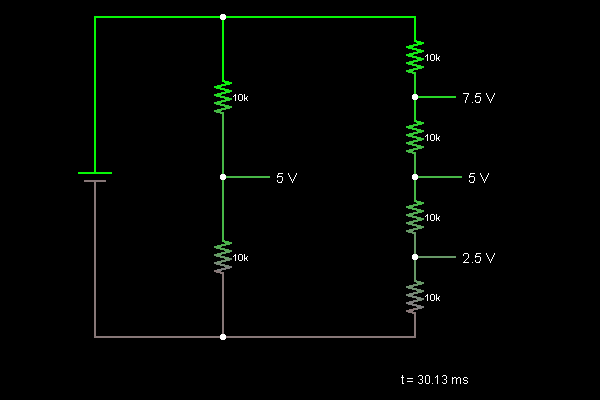# Circuit Simulator - Voltage Divider

## Where can I get Voltage Divider Circuit Diagram with Explanation?

IndiaBIX provides you lots of fully solved Voltage Divider circuit diagram with detailed explanation and working principles.

## How to design a Voltage Divider (electronic circuit)?

You can easily design the Voltage Divider circuit by practicing the exercises given below. Here you can design and simulate your own electronic circuits with this Online Circuit Designer and Simulator.### Circuit Description:

This is a voltage divider, a simple circuit that can be used to derive a reference voltage from a known supply voltage. In the middle, two equal resistors generate a 5 V voltage from the 10 V supply. On the right, four resistors provide 7.5 V, 5 V, and 2.5 V. -- Credits: Mr. Paul Falstad.

 Babu said: (Nov 1, 2010) This simulation gives more idea.

 Vicky said: (Aug 2, 2012) I have a doubt. Two voltage sources connected in parallel then what happen. Please give the answer.

 Shadow said: (Aug 13, 2012) Hey. If two volatge sources connected in parallel then the current flow will be the same.

 Thashu said: (Aug 25, 2012) Give me clear information of the thevinin's and norton's theorem.

 Chaitra said: (Oct 28, 2012) How many resistors should we use to divide 5 volts into 1 volts across each resistor? Is it 5?

 Sourabh said: (Nov 4, 2012) How to use of thevenin theorem in practical?

 Vinodkumar said: (Feb 6, 2013) How to generate +2.5 and -2.5 voltages from single +3 Volts Battery?

 Million said: (Apr 16, 2013) I need brief explanation about mesh analysis of current and voltage.

 Vis Lin said: (Nov 6, 2014) How to build a connection of 12 AC Fan(220~240V;0.09A)?